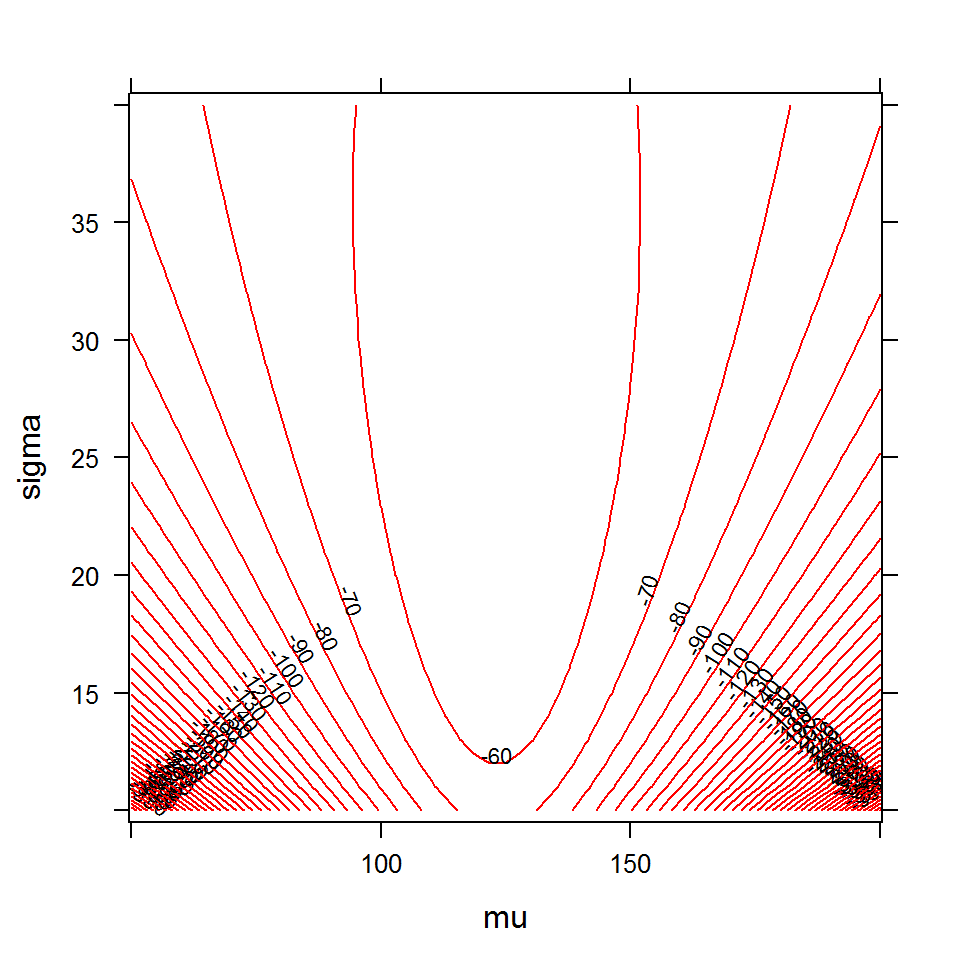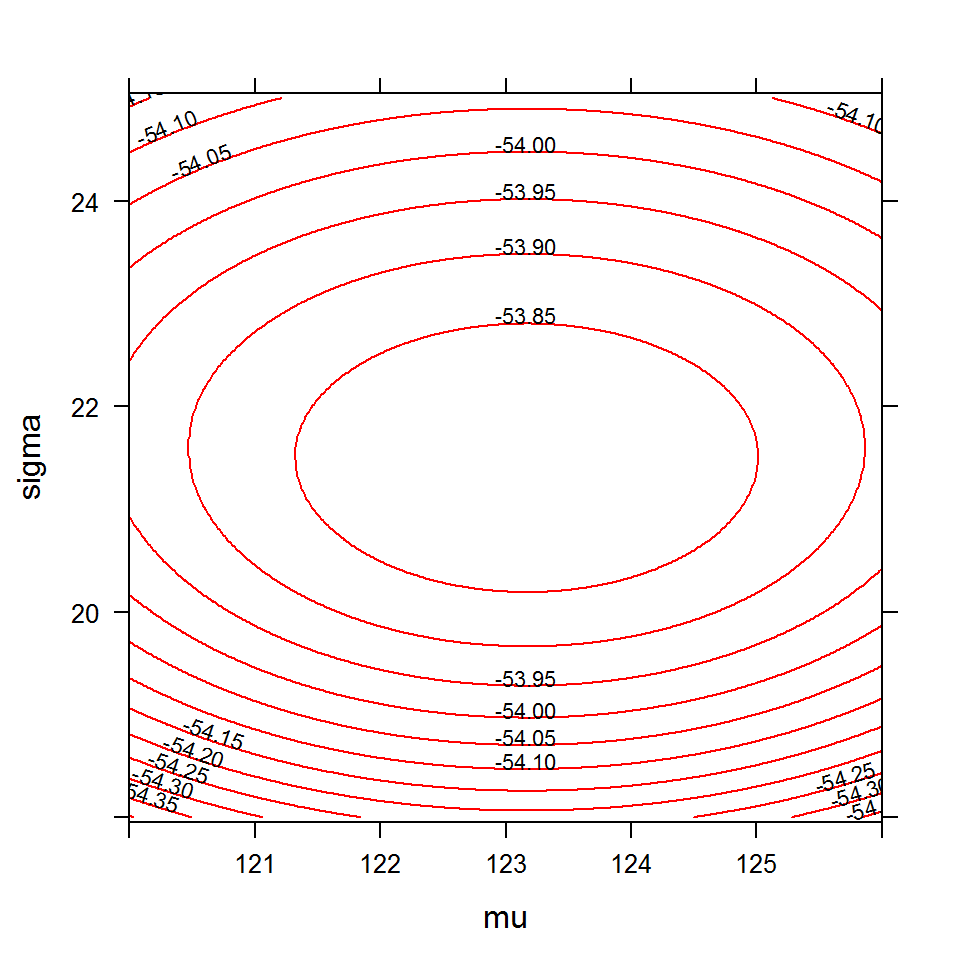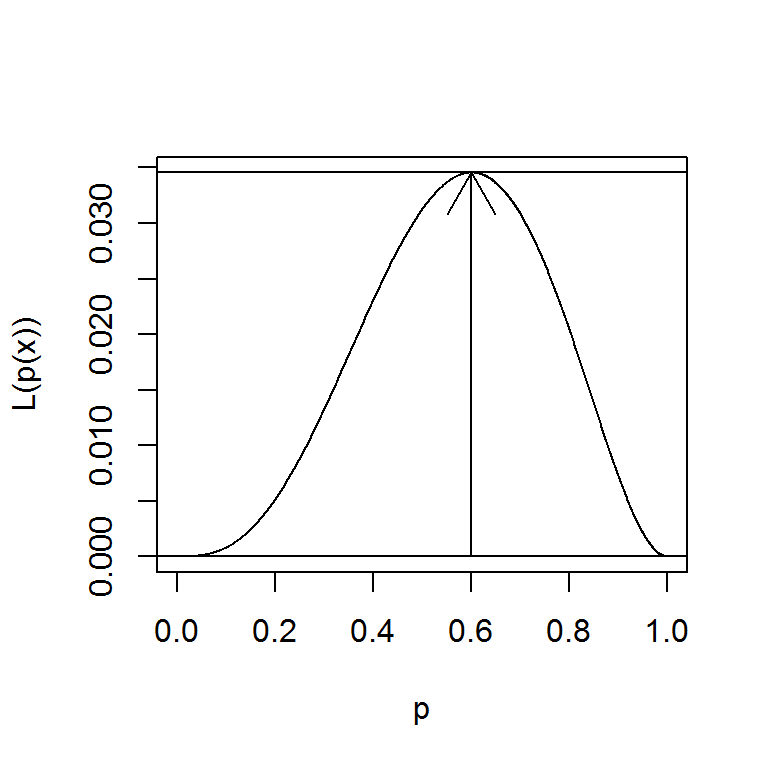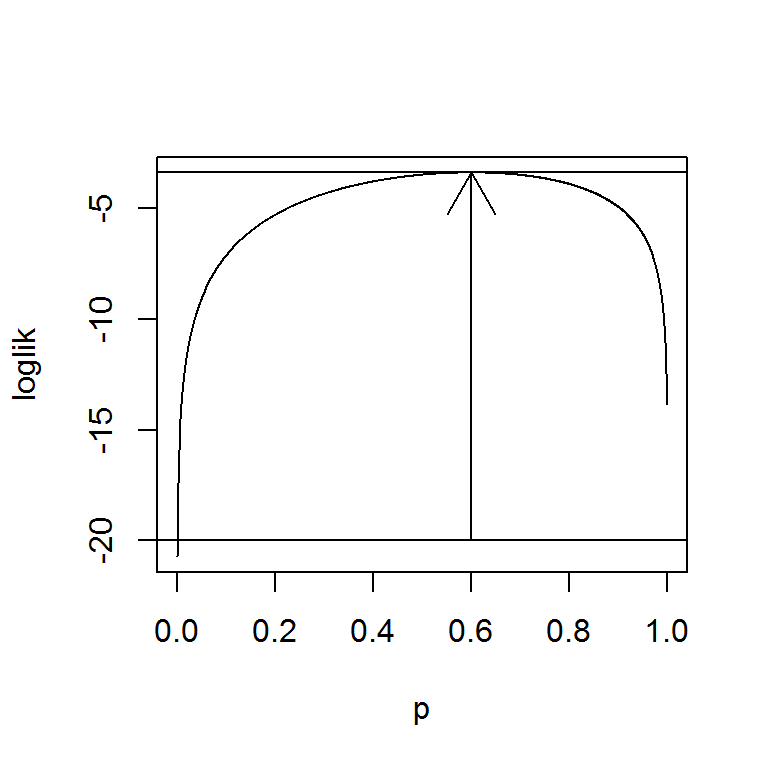### MAXIMUM LIKELIHOOD ESTIMATION (MLE):

Specific derivations can be found here.

From this post:

We want to estimate the mean and variance of the stem diameters (in mm) of Pinus radiata trees based on twelve observations, and using a normal model:

The point of departure is the pdf of the normal distribution:

$f_X(x \vert \mu,\sigma) = \frac{1}{\sqrt{2\pi}\,\sigma}\exp{\frac{-(x-\mu)^2}{2\sigma^2}}$

Wikipedia Now we look at this function from a different perspective by considering the observed values $$x_1, x_2,\dots, x_n$$ to be fixed “parameters” of this function, whereas $$\theta$$ will be the function’s variable and allowed to vary freely; this same function will be called the likelihood:

$\mathcal L( \mu,\sigma ; x_i)=\prod_{i=1}^n f_X(x_i \vert \mu, \sigma)=\prod_{i=1}^n \frac{1}{\sqrt{2\pi}\,\sigma}\exp{\frac{-(x_i-\mu)^2}{2\sigma^2}}$

Here the observed values $$x_i$$ are fixed, and the function depends on the parameters $$\mu$$ and $$\sigma$$. The idea is to maximize the value of the formula. However, multiplying small fractions becomes numerically unstable. In comes the log-likelihood function:

the log-likelihood:

$\ln \mathcal {L}(\theta \,;\,x_{1},\ldots ,x_{n})=\sum _{i=1}^{n}\ln f_X(x_{i}\vert \theta )$

The average log-likelihood function is denoted as $$\hat l$$:

$\hat l=\frac {\ln \mathcal L}{n}$

The method of maximum likelihood estimates by finding a value of $$\theta$$ that maximizes $$\hat l (\theta ;x)$$. This method of estimation defines a maximum likelihood estimator (MLE) of $$\theta$$:

$\{\hat \theta_{\text{mle}}\} \subseteq \{\underset{\theta \in \Theta }{\operatorname{arg}\text{max}}\ \hat {l}(\theta \,;\,x_{1},\ldots ,x_{n})\}$

In many instances, there is no closed form, and an computational or iterative procedures will be used. Here is a link to Andrew Ng’s gradient descent will be used.

\begin{align}\log \mathcal L( \mu,\sigma ; x_i) &= \sum_{i=1}^n \log\left(\frac{1}{\sqrt{2\pi}\,\sigma}\exp{\frac{-(x_i-\mu)^2}{2\sigma^2}}\right)\\ &= \sum_{i=1}^n\log\left( \frac{1}{\sqrt{2\pi}\,\sigma}\right)+\sum_{i=1}^n\log\left(\exp{\frac{-(x_i-\mu)^2}{2\sigma^2}}\right)\\ &= \sum_{i=1}^n\log\left( (2\pi)^{-1/2}\right) + \sum_{i=1}^n \log\left( (\sigma)^{-1}\right)+\sum_{i=1}^n\log\left(\exp{\frac{-(x_i-\mu)^2}{2\sigma^2}}\right)\\ &=-\frac{n}{2}\log(2\pi)-n\log(\sigma)-\frac{1}{2\sigma^2}\sum_{i=1}^n(x_i - \mu)^2 \end{align}

Now taking the partial derivative with respect to $$\mu$$:

$\frac{\partial \log L}{\partial \mu}= \frac{1}{2\sigma^2} 2 \sum_{i=1}^n(x_i - \mu)$

and setting it to zero:

$0 = \sum_{i=1}^n(x_i - \mu)$

$\mu = \frac{\sum_{i=1}^nx_i}{n}$

Actually, this is the mean, and it is the sample mean providing and estimation of $$\mu$$ (i.e. $$\hat \mu$$).

We can also take the partial with respect to $$\sigma$$:

\begin{align}\frac{\partial \log L}{\partial \sigma}&= -\frac{n}{\sigma}+\frac{2}{2}\sum_{i=1}^n(x_i - \mu)^2 \sigma^{-3}\\ &=-\frac{n}{\sigma}+\frac{1}{\sigma^3}\sum_{i=1}^n(x_i - \mu)^2\\ \end{align}

And setting it to zero:

$0 = -\frac{n}{\sigma}+\frac{1}{\sigma^3}\sum_{i=1}^n(x_i - \mu)^2$

Hence,

$\sigma^2 =\frac{\sum_{i=1}^n (x_i - \mu)^2}{n}$

which is really a biased estimate of the variance in the population, $$\sigma$$ (i.e. $$s^2$$). This bias arises because maximum likelihood estimates do not take into account the loss of degrees of freedom when estimating fixed effects.

diams = c(150, 124, 100, 107, 170, 144,
113, 108, 92, 129, 123, 118)

# A simple function to calculate log-likelihood.
# Values will be easier to manage
loglike = function(data, mu, sigma) {
loglike = 0
for(obs in data){
loglike = loglike +
log(1/(sqrt(2*pi)*sigma) *
exp(-1/2 * (obs - mu)^2/(sigma^2)))
}
return(loglike)
}
# Let's try some combinations of parameters and
# plot the results
params = expand.grid(mu = seq(50, 200, 1),
sigma = seq(10, 40, 1))
params$logL = with(params, loglike(diams, mu, sigma)) summary(params) ## mu sigma logL ## Min. : 50 Min. :10 Min. :-420.42 ## 1st Qu.: 87 1st Qu.:17 1st Qu.: -89.43 ## Median :125 Median :25 Median : -69.39 ## Mean :125 Mean :25 Mean : -85.58 ## 3rd Qu.:163 3rd Qu.:33 3rd Qu.: -59.13 ## Max. :200 Max. :40 Max. : -53.81 library(lattice) contourplot(logL ~ mu*sigma, data = params, cuts = 30, col=2, labels = list(cex = 0.7))# Zooming in params = expand.grid(mu = seq(120, 126, 0.01), sigma = seq(18, 25, 0.1)) params$logL = with(params, loglike(diams, mu, sigma))
summary(params)
##        mu            sigma           logL
##  Min.   :120.0   Min.   :18.0   Min.   :-54.40
##  1st Qu.:121.5   1st Qu.:19.7   1st Qu.:-54.03
##  Median :123.0   Median :21.5   Median :-53.93
##  Mean   :123.0   Mean   :21.5   Mean   :-53.96
##  3rd Qu.:124.5   3rd Qu.:23.3   3rd Qu.:-53.87
##  Max.   :126.0   Max.   :25.0   Max.   :-53.81
contourplot(logL ~ mu*sigma, data = params, cuts = 10, col=2,
labels = list(cex = 0.7))# Compare to:

mean(diams); sd(diams)
##  123.1667
##  22.38438

From here.

##### ML for Bernoulli trials

If our experiment is a single Bernoulli trial and we observe $$X = 1$$ (success) then the likelihood function is $$\mathcal L(p ; x) = p$$ . This function reaches its maximum at $$\hat p =1$$. If we observe $$X = 0$$ (failure) then the likelihood is $$\mathcal L(p ; x) = 1 − p$$, which reaches its maximum at $$\hat p=0$$. Of course, it is somewhat silly for us to try to make formal inferences about $$\theta$$ on the basis of a single Bernoulli trial; usually multiple trials are available.

Suppose that $$X = (X_1, X_2, \dots, X_n)$$ represents the outcomes of $$n$$ independent Bernoulli trials, each with success probability $$p$$. The likelihood for $$p$$ based on $$X$$ is defined as the joint probability distribution of $$X = (X_1, X_2, \dots, X_n)$$. Since $$X = (X_1, X_2, \dots, X_n)$$ are iid random variables, the joint distribution is

\begin{align}\mathcal L(\theta;x) &=\prod_{i=1}^{n}f_X(x_i;\theta)=\prod_{i=1}^{n} \theta^x_i(1−\theta)^{1−x}\\[2ex] &=\theta^{x_1}(1-\theta)^{1-x_1}\dots\theta^{x_n}(1-\theta)^{1-x_n}\tag{1}\\ \end{align}

$$(1)$$ just reflects the probability mass function of the Bernouilli distribution.

For the log-likelihood,

\begin{align}\ln \mathcal L(\theta ; x)&= \ln \theta \left(\sum_{i=1}^n x_i\right) + \ln(1- \theta)\left(n -\sum_{i=1}^n x_i\right)\\[2ex] &=n\bar x \ln \theta + n(1- \bar x)\ln(1- \theta) \end{align}

Differentiating and setting it up to zero,

\begin{align} \frac{\partial}{\partial \theta} \ln \mathcal L(\theta; x) &= n\left(\frac{\bar x}{\theta}-\frac{1-\bar x}{1-\theta}\right) \\[2ex] &=\frac{\bar x (1-\theta) - \theta(1 - \bar x)}{\theta (1-\theta)}=0 \end{align}

Therefore $$\hat \theta = \bar x$$

##### ML for Binomial trials:

Suppose that is an observation from a binomial distribution, $$X \sim Bin(n, p )$$, where $$n$$ is known and $$p$$ is to be estimated. The likelihood function is

$\mathcal L(p;x)=\frac{n!}{x!(n−x)!}p^x(1−p)^{n−x}$

which, except for the factor $$\frac{n!}{x!(n−x)!}$$, is identical to the likelihood from $$n$$ independent Bernoulli trials with $$x=\sum_{i=1}^n x_i$$. But since the likelihood function is regarded as a function only of the parameter p, the factor $$\frac{n!}{x!(n−x)!}$$ is a fixed constant and does not affect the MLE. Thus the MLE is again $$\hat p = x/n$$, the sample proportion of successes.

You get the same value by maximizing the binomial loglikelihood function:

$l(p;x)=k+x \log p+(n−x) \log(1−p)$

where $$k$$ is a constant that does not involve the parameter $$p.$$

Let’s see what the likelihood function looks like with a binomial experiment yielding $$3 \text{ heads}$$ and $$2 \text{ tails}:$$

p = seq(0, 1, 0.001)
L = p^3 * (1 - p)^2
plot(p, L, type = "l", xlab="p", ylab="L(p(x))")
max(L)
##  0.03456
d = cbind(p,L)
(MLE = d[which.max(d[,2]), 1])
##   p
## 0.6
arrows(x0 = MLE, y0 = 0, x1 = MLE, y1 = max(L))
abline(h = 0); abline(h = max(L))# As for the log-likelihood:

loglik = 3 * log(p) + 2 * log(1 - p)
plot(p, loglik, type = "l")
d = cbind(p,loglik)
MLE = d[which.max(d[,2]), 1]
arrows(x0 = MLE, y0 = -20, x1 = MLE, y1 = max(loglik))
abline(h = -20); abline(h = max(loglik))##### Linear Regression:

The model is:

$y_i = \alpha + \beta x_i + \epsilon_i$

Because the errors are independent with mean of $$0$$ and unknown variance $$\sigma^2:$$

\begin{align}\mathcal L(\alpha, \beta, \sigma^2; x, y)&=f_X(y_1\vert \alpha + \beta x_1, \sigma^2)\quad f_X(y_2\vert \alpha + \beta x_2, \sigma^2)\quad\dots\quad f_X(y_n\vert \alpha + \beta x_n, \sigma^2)\\[2ex] &= \small \frac{1}{\sigma \sqrt{2\pi}}\exp{-\frac{(y_1 - \alpha -\beta x_1)^2}{2\sigma^2}}\frac{1}{\sigma \sqrt{2\pi}}\exp{-\frac{(y_2 - \alpha -\beta x_2)^2}{2\sigma^2}}\dots \frac{1}{\sigma \sqrt{2\pi}}\exp{-\frac{(y_n - \alpha -\beta x_n)^2}{2\sigma^2}}\\[2ex] &=\frac{1}{\sqrt{(2\pi\sigma^2)^n}}\exp -\frac{1}{2\sigma^2}\sum_{i=1}^n \left(y_i - (\alpha + \beta x_i\right))^2 \end{align}

The log-likelihood:

$\ln \mathcal L(\alpha,\beta,\sigma^2; x,y)=-\frac{n}{2}\ln 2\pi\sigma^2 \color{red}{-} \frac{1}{2\sigma^2}\sum_{i=1}^n(y_i -(\alpha + \beta x_i))^2$

So when the residuals are minimum (i.e. $$\sum_{i=1}^n(y_i -(\alpha + \beta x_i))^2$$), the log-likelihood will be maximized (due to the negative sign).

Since OLS minimizes residuals, it will correspond to the MLE, provided the errors are following a normal distribution around the mean (the predicted values).

We can estimate the slope $$\beta$$ for instance:

\begin{align}\frac{\partial\ln \mathcal L(\alpha,\beta,\sigma^2; x,y)}{\partial \beta}&= \frac{1}{\sigma^2}\sum_{i=1}^nx_i(y_i -(\alpha + \beta x_i)) \end{align}

and setting to zero:

\begin{align}0&= \frac{1}{\sigma^2}\sum_{i=1}^nx_i(y_i -(\alpha + \beta x_i))\\ 0&= \color{blue}{\sum_{i=1}^nx_i(y_i -(\alpha + \beta x_i))} \end{align} \begin{align}0&= \sum_{i=1}^n x_i y_i - x_i \alpha - \beta x_i^2))\\ \sum_{i=1}^n\beta x_i^2 &= \sum_{i=1}^n x_i y_i - x_i \alpha \end{align}

Therefore,

$\hat \beta = \frac{\sum_{i=1}^n x_i y_i - x_i \alpha }{\sum_{i=1}^n x_i^2}$

Now the $$\sum_{i=1}^n(y_i -(\alpha + \beta x_i))$$ is the estimated error, and

$\color{blue}{0=\sum_{i=1}^nx_i(y_i -(\alpha + \beta x_i))}$

one of the conditions of classical OLS.